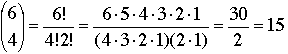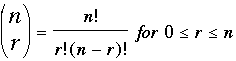SEARCH HOMEMath Central Quandaries & QueriesQuestion from Erin, a student: You have enough money to buy 4 books at the book fair. There are 6 books to choose from. How many different combinations are there.Hello,
The number of possible combinations is given by:In general, the number of combinations of size   r  from a collection of size  n  is given by:TylerMath Central is supported by the University of Regina and The Pacific Institute for the Mathematical Sciences.